Courses

# Test: Quantum Numbers & Electronic Configuration

## 20 Questions MCQ Test Chemistry for JEE | Test: Quantum Numbers & Electronic Configuration

Description
This mock test of Test: Quantum Numbers & Electronic Configuration for JEE helps you for every JEE entrance exam. This contains 20 Multiple Choice Questions for JEE Test: Quantum Numbers & Electronic Configuration (mcq) to study with solutions a complete question bank. The solved questions answers in this Test: Quantum Numbers & Electronic Configuration quiz give you a good mix of easy questions and tough questions. JEE students definitely take this Test: Quantum Numbers & Electronic Configuration exercise for a better result in the exam. You can find other Test: Quantum Numbers & Electronic Configuration extra questions, long questions & short questions for JEE on EduRev as well by searching above.
QUESTION: 1

### Direction (Q. Nos. 1-10) This section contains 10 multiple choice questions. Each question has four choices (a), (b), (c) and (d), out of which ONLY ONE option is correct. Q. Which is a possible set of quantum numbers for a valence unpaired electrons in ground state atom of phosphorus (Z = 15)?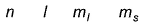Solution: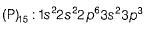Valence unpaired electrons are in 3p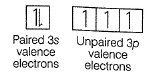Valence unpaired electrons are in 3p.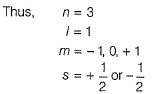Thus, (d).

QUESTION: 2

### For a multi-electron atom, set of quantum numbers is given as 2,0,0,1/2 ; 2,0,0,-1/2 Q. Thus, the next higher allowed set of n and / quantum numbers for this atom in its ground state is

Solution:

Given a set of quantum numbers, n=2,l=0 for a multi-electron atom refers to 2s orbital.

The next higher allowed set of 'n' and 'l' quantum numbers for this atom in the ground state is n=2,l=1. This corresponds to 2p orbital.

Note: The orbital with a higher value of the sum (n+l) has higher energy.

For 2s orbital (n+l)=(2+0)=2

For 2p orbital (n+l)=(2+1)=3

QUESTION: 3

### Magnetic moments of the following isoelectronic species (24 electrons) are in order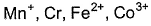Solution: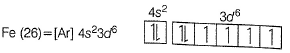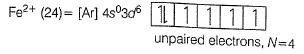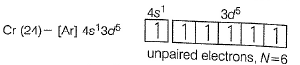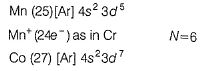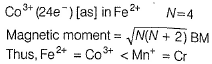QUESTION: 4

The orbital angular momentum for a d-orbital electron is given by

Solution: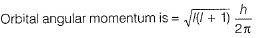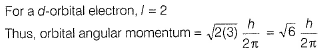QUESTION: 5

The number of radial nodes in 3s and 2p respectively are

Solution:

Radial nodes = (n - l - 1)
Angular nodes = l, total nodes = (n - 1), nodal plane = l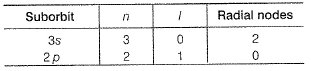QUESTION: 6

A hydrogen like species in fourth orbit has radius 1.5 times that of Bohr's orbit. In neutral state, its valence electron is in

Solution: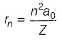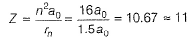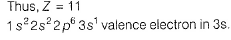QUESTION: 7

Following suborbits with values of n and l are given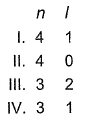Q. Increasing order of energy of these suborbits is

Solution: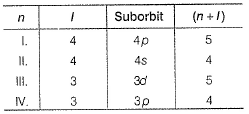By Aufbau rule, smaller the value of (n + I), smaller the energy. If (n + I) is same for two or more orbits, suborbit with lower value of n, has smaller energy.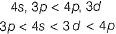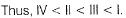QUESTION: 8

The correct set of four quantum numbers for the valence electron of rubidium atom (Z = 37) is

[JEE Main 2013]

Solution: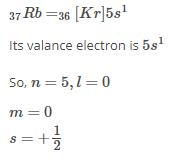QUESTION: 9

For Cr(24), (EC) : [Ar] 4s1 3d5 The number of electrons with l = 1 to l = 2 are respectively

Solution:

EC of Cr (24) is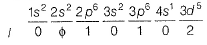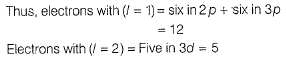QUESTION: 10

Last filling electron in lanthanides find place in 4f-orbital. Which of the following sets of quantum number is correct for an electron in 4f orbital?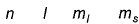Solution:

4l -orbital
Thus, n = 4, l = 3
m, (anyone) = - 3, - 2, - 1, 0, +1, + 2, + 3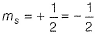*Multiple options can be correct
QUESTION: 11

Direction (Q. Nos. 11-15) This section contains 5 multiple choice questions. Each question has four choices (a), (b), (c) and (d), out of which ONE or  MORE THANT ONE  is correct.

Q. Quantum numbers have been matched with their characteristics. Select correct matching(s).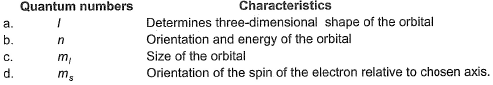Solution:

(a) Three-dimensional shape of orbital is derived from angular quantum number: True
(d) Spin of the electron is derived from spin quantum number: True

QUESTION: 12

In the following pair, each has two orbitals I and II. Select the ones in which II experiences larger effective nuclear charge than l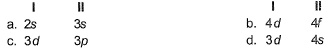Solution:

Nuclear charge is defined as the net positive charge experienced by an electron in the orbital of a multi-electron atom. The closer the orbital, the greater is the nuclear charge experienced by the electrons in it.

Since 3p is closer to nucleus than 3d so it will experience greater Z

Hence C

*Multiple options can be correct
QUESTION: 13

In a multi-electron atom, which of the following orbitals described by the three quantum numbers will have the same energy in the absence of magnetic and electric fields?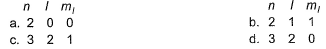Solution:

(a) 2s (b) 2 p (c) 3d (d) 3d
Thus, (c) and (d), both 3d, have equal energy.

*Multiple options can be correct
QUESTION: 14

Which of the following statements is/are correct?

Solution:

(a) Cr (24) = [Ar] 4s13d5 Correct
(b) K = 1s22s22 p63s23p64s1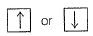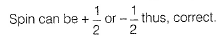(c) p-suborbit has three orbitals, each orbital can have maximum of two electrons thus, correct.
(d) s-suborbit has one orbital and this orbital can have maximum of two electrons thus, correct.

QUESTION: 15

The quantum number which specifies the location of an electron as well as energy is

Solution:

According to the given sets of options, only option d is correct. The reason or exceptional configuration of Cr is stability of half-filled d subshell. If the Aufbau principle was not followed then the configuration of Cr be [Ar] 3d6. So, if the Aufbau principle won’t be followed, then the options won’t have an electron in their 4s subshell.

QUESTION: 16

Quantum Numbers are solutions of _____________

Solution:

When the wave function for an atom is solved using the Schrodinger Wave Equation, the solutions obtained are called the Quantum Number which are basically n, l and m.

QUESTION: 17

Which quantum numbers gives the shell to which the electron belongs?

Solution:

The principal quantum number, n, gives the shell to which the electron belongs. The energy of the shell is dependent on ‘n’.

QUESTION: 18

Direction (Q. Nos. 18) Choice the correct combination of elements and column I and coloumn II  are given as option (a), (b), (c) and (d), out of which ONE option is correct.

Q. Match the entries in Column I with correctly related quantum number(s) in Column II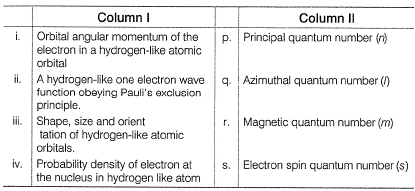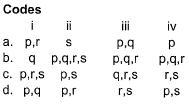Solution:

(i) Orbital angular momentum L =
L depends on the value of l (azimuthal quantum number)
(ii) To describe wave function (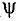), n, I and m are needed, if, it obeys Pauli's exclusion principle, then s is also needed.
(iii) Value of n, I and m are needed to determine size, shape and orientation.
(iv) Probability density (2) is based on n, I and m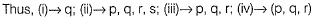QUESTION: 19

Direction (Q. Nos. 19 and 20) This section contains 2 questions. when worked out will result in an integer from 0 to 9 (both inclusive)

Q.   Bond order of 1.5 is shown by

Solution:

MO configuration of O2+ (8+8-1=15)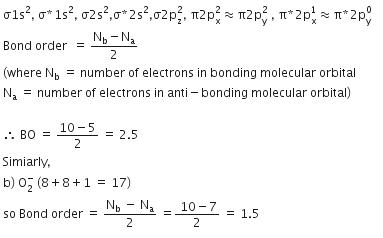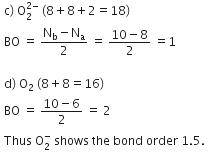*Answer can only contain numeric values
QUESTION: 20

The maximum number of electrons that can have principal quantum number, n = 3 and spin quantum number,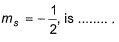Solution:

(9) n = 3 can have suborbits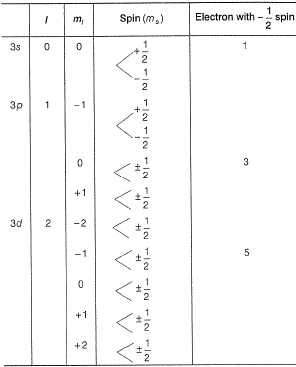Total nine electrons.